# Speculation Regarding Quantum Transitions

The contents of this "Ramblings Appendix" chapter are pure speculation. I am definitely not an authority on quantum mechanics, but I have for some time been interested in interpretations of the wave function. It is this, along with my quantitative interests in digital holography, that have led to the set of thoughts presented below.

## Digital Holography

When a ray of coherent, monochromatic light passes through a square aperture, a specific diffraction pattern is created. The same result is achieved by bouncing the light off of one side of a cube [serving as the square aperture]. In this manner, information about a localized structure (the aperture) is preserved in a (diffraction) pattern that formally extends to infinity. A hologram is created by "storing" the diffraction pattern (amplitude with no phase) as an image.

This process can be reversed. A ray of coherent, monochromatic light that bounces off of (or shines through) the holographic image will — at the appropriate distance from the hologram — display an image of the original compact aperture.

Note that, either way — that is, whether the aperture is being used to create the diffraction pattern or vise versa — the diffraction pattern/hologram can be viewed as a probability distribution.

## Quantum Wavefunction

### First Thoughts

This sounds suspiciously like photoemission or an atomic transition:

1. Let's say that when an electron is bound to a nucleus in a particular energy state, this state is represented by a "surface" — perhaps it should be a more volume-filling structure — whose multiple-aperture/grid structure (each subgrid square aperture having a different, specified opacity) both appropriately represents the wavefunction and defines a hologram. In this manner, when an electron is bound to an atomic nucleus, information regarding its position/momentum is viewed as a wave function (probability distribution).
2. PHOTOIONIZATION:   When a photon (of the proper frequency) strikes the atom, it can react with the wavefunction in such a manner that it ejects the electron. That is to say, the result of the light passing through (bouncing off of) the wavefunction (hologram) is to form a compact entity (the electron) that is moving away from the atomic nucleus.
1. Note that if the incident ray of light has an incorrect frequency and/or hits the hologram at an incorrect angle, the resulting diffraction pattern does not generate the compact entity (electron). But it is natural to expect that the likelihood that the photon hits with the correct angle of incidence will be higher for axisymmetric wavefunctions (holographic surface) and will be even higher in the case of spherical symmetry.
2. The direction the electron gets ejected should naturally be at a well-defined angle with respect to the direction of incidence of the initial ray of light (photon).
3. EXCITATION (BOUND-BOUND TRANSITION):   Perhaps the holographic surface is flexible, permitting it to undergo oscillations. The eigenfunctions corresponding to various modes of oscillation might in some way be associated with the holograms that represent other acceptable bound orbital levels. Then if the frequency of an incident photon resonates with one mode's associated oscillation eigenfrequency, the holographic structure could change to indicate that the electron has moved to a different orbital level.

Also, it "explains" why the atomic state cannot be measured without necessarily altering the state.

### Second Thoughts

Keep in mind that a free electron or a free photon is represented by a wave packet — see Wikipedia's graphical illustration. "Depending on the wave equation, the wave packet's profile may remain constant (no dispersion) or it may change (dispersion) while propagating."

So, assuming that the hologram — e.g., apertures cut out of a thin spherical shell — is stationary, the incoming photon that strikes the hologram will be represented by a certain well-defined, traveling wave packet and, if ionization is successfully achieved, the outgoing electron will be represented by a different (but, still, a certain well-defined) traveling wave packet.

A subsection of a wikipedia article titled, Visualizing the hydrogen electron orbitals, displays a cross-sectional image of the probability distributions of six separate electron orbitals.

## Characteristic Size and Spin

What is the angular momentum of a spherical shell spinning with angular frequency,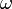$~\omega$, whose radius is the Bohr radius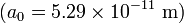$~(a_0 = 5.29 \times 10^{-11}~\mathrm{m})$ and whose mass is one electron mass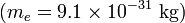$~(m_e = 9.1\times 10^{-31} ~\mathrm{kg})$? Compare this to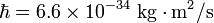$~\hbar = 6.6 \times 10^{-34}~\mathrm{kg} \cdot \mathrm{m}^2/\mathrm{s}$. Drawing from Wikipedia, the relevant moment of inertia is,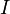$~I$$~=$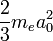$~\frac{2}{3} m_e a_0^2$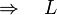$~\Rightarrow ~~~L$$~=$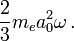$~\frac{2}{3} m_e a_0^2 \omega \, .$

What is the spin period if we set this angular momentum to half of the Planck constant?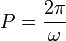$~P = \frac{2\pi}{\omega}$$~=$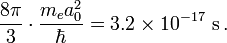$~\frac{8\pi}{3}\cdot \frac{m_e a_0^2}{\hbar} = 3.2 \times 10^{-17}~\mathrm{s} \, .$

For comparison, what is the light-travel time across the sphere?$~t$$~=$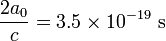$~\frac{2a_0}{c} = 3.5 \times 10^{-19} ~\mathrm{s}$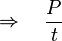$~\Rightarrow ~~~ \frac{P}{t}$$~=$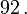$~92 \, .$